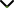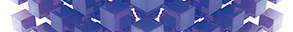# Discrete Fractional-Order Systems with Applications in Engineering and Natural Sciences

Publishing date
01 Mar 2021
Status
Closed
30 Oct 2020

Guest Editors

1Suez Canal University, Ismailia, Egypt

2King Saud University, Riyadh, Saudi Arabia

3Mansoura University, Mansoura, Egypt

4Shandong Univeristy of Science and Technology, Qingdao, China

5Prince Sattam Bin Abdulaziz University, Al-Kharj, Saudi Arabia

This issue is now closed for submissions.
More articles will be published in the near future.

# Discrete Fractional-Order Systems with Applications in Engineering and Natural Sciences

This issue is now closed for submissions.
More articles will be published in the near future.

## DescriptionFractional order differentiation and integration can be considered as a generalization of conventional integer order calculus to non-integer real or even complex-valued orders. The history of fractional calculus has started about 300 years ago. Fractional calculus can describe memory dependent behaviours and inherited properties of nonlinear systems. In the past few decades, employing fractional calculus (FC) in mathematical modelling of some engineering, physical, and economic systems represented an optimal choice for the formulation of more realistic models. Recently, FC has proved itself as a useful tool for applications in many fields of research such as physical systems, biomedicine, nonlinear electronic circuits, chaos-based cryptography, and image encryption. Examples of systems that can be precisely described by fractional-order differential equations (FODEs) involve viscoelastic material models, electrical components, electronic circuits, diffusion waves, propagation of waves in nonlocal elastic continua, hydrologic systems, earthquakes’ nonlinear oscillations, models of world economies, and equations of muscular blood vessels. In general, FODEs are more appropriate than the corresponding classical integer-order mathematical models in systems where memory effects are crucial, such as thermodynamics, chemistry, control theory, economic, biological, and social models.

Furthermore, the first one is continuous fractional-order calculus, which mainly corresponds to the integer-order differential calculus. The other one is the discrete fractional-order calculus, which mainly corresponds to the integer-order difference calculus. As an extension of the integer-order difference equation, the fractional-order difference equation has the function of long memory. The fractional difference equation, named discrete fractional equation, also can be regarded as the discrete form of a continuous fractional differential equation. The specific field of discrete fractional calculus (DFC) is a hot topic which develops rapidly in recent years. More recently, chaotic discrete fractional dynamical systems were proved their efficiency in some modern chaos based encryption schemes.

The Special Issue aims to gather results or studies on developments such as the novel discrete fractional order in engineering, physical, biological, and economical models, analytical insights into such models, stability analysis, bifurcation analysis, determination of chaotic behaviour, and implementation of chaos control methods. We welcome original research articles as well as review papers with a focus on the advances of discrete fractional-order systems with applications in engineering and natural sciences.

Potential topics include but are not limited to the following:

• Development discrete fractional-order modelling in engineering and natural sciences
• Dynamics of discrete fractional-order systems in engineering and natural sciences
• Design and realization of discrete fractional order differentiators and integrators
• Design and realization of discrete fractional order circuits including generalized filters and oscillators
• Highly efficient information processing and artificial intelligence applications
• Chaotic discrete fractional-order secure communication schemes
• Chaotic image encryption schemes based on discrete fractional-order systems
• Bifurcation and chaos analysis of discrete fractional-order models
• Chaos control strategies for these models
• Memristor-based discrete fractional-order systems
• Chaos synchronization of discrete fractional-order models in relevant areas of engineering and natural sciencesJournal metrics
Acceptance rate27%
Submission to final decision64 days
Acceptance to publication34 days
CiteScore1.800
Journal Citation Indicator0.400
Impact Factor1.305Author guidelinesEditorial boardDatabases and indexing

Article of the Year Award: Outstanding research contributions of 2020, as selected by our Chief Editors. Read the winning articles.